EXPERIMENT NO 9.

Binomial array

Practical Session Plan

 Time ( min) Content Learning Aid / Methodology Faculty Approach Typical Student Activity Skill / Competency Developed 10 Relevance and significance of experiment Chalk & Talk , Presentation Introduces, Facilitates, Monitors Listens, Participates, Discusses Knowledge, Communication, intrapersonal 20 Explanation of experiment Chalk & Talk , Presentation Introduces, Facilitates, Explains Listens Knowledge, Communication, intrapersonal, Application 10 Calculations N/A Explains, Monitors Participates, Discusses Knowledge, Communication, Intrapersonal, Application 50 Programming Demonstration Explains, Monitors Participates, Discusses Debate, comprehension, Hands on experiment 10 Debugging & Simulating Demonstration Explains, Monitors Participates, Discusses Hands on experiment 10 Results and conclusions Keywords Lists, Facilitates Listens, Participates, Discusses Knowledge, Communication, intrapersonal, Comprehension 10 Oral Question Discussion Discussion Discussion Identifies & Participates Knowledge Intrapersonal

Title: Binomial array antenna

Objectives: Model the Binomial array to analyze the performance parameters

Aim:

Â· To sketch the radiation pattern of Binomial array with uniform spacing & amplitude

• To compute performance parameters

Tool used: MATLAB.

Theory:

Binomial Array

Binomial array are conceptually very simple. Also, binomial array doesnâ€™t have any side-lobes in general. But, these arrays suffer from very poor aperture efficiency and highly tapered distribution. So, binomial arrays are not preferable for large arrays. Array excitation corresponding to binomial array of N elements is given by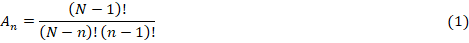Array factor corresponding to the above excitation is given as,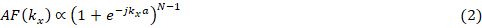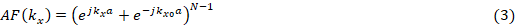Reasons for choosing the above array factor are:

Â· Array factor should be a periodic function in kx domain with a period of 2Ï€/a.

Â· Array factor should have an order of N-1 indomain.

Â· All the zeros should present at one single location, i.e., kx = kx0, so that array factor will not have any side-lobes

For synthesizing such an array, one can apply either the Fourier transform or the definition of array factor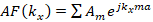itself. The method to evaluate the array coefficients corresponding to binomial array is explained by using the following example.

The array factor for the binomial array is represented by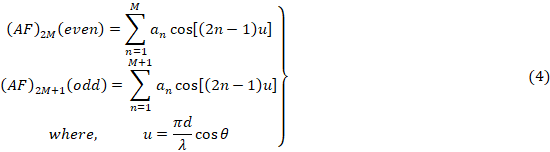where the anâ€™s are the excitation coefficients which will now be derived.

Excitation Coefficients

To determine the excitation coefficients of a binomial array, J. S. Stone suggested that the function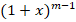be written in a series, using the binomial expansion, as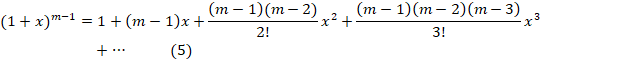The positive coefficients of the series expansion for different values of mare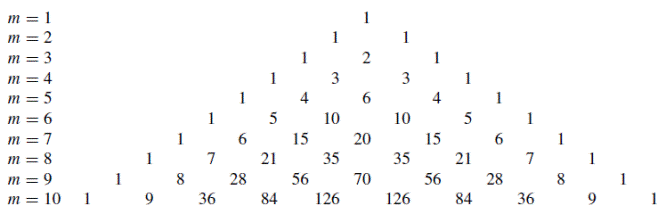The above represents Pascalâ€™s triangle. If the values of m are used to represent the number of elements of the array, then the coefficients of the expansion represent the relative amplitudes of the elements. Since the coefficients are determined from a binomial series expansion, the array is known as a binomial array.

The amplitude coefficients for the following arrays are:

1. Two elements (2M = 2)

a1 = 1

2. Three elements (2M + 1 = 3)

2a1 = 2 âž± a1 = 1

a2 = 1

3. Four elements (2M = 4)

a1 = 3

a2 = 1

4. Five elements (2M + 1 = 5)

2a1 = 6 âž± a1 = 3

a2 = 4

a3 = 1

The coefficients for other arrays can be determined in a similar manner.

Design Procedure

One of the objectives of any method is its use in a design. For the binomial method, as for any other non-uniform array method, one of the requirements is the amplitude excitation coefficients for a given number of elements. This can be accomplished using either (5)

Algorithm:

1. Read the values of n, f and d.

2. Calculate Î»,Î²,Ïˆ.

3. Read array coefficients(at least 5) in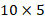matrix.

4. Calculate x=modulus(N,2)

5. If x=0 then used6. If not,Where,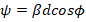7. Calculate absolute value AF.

8. Also calculate normalized value AF.9. Plot Ï• vs Ï‰.

10. Calculate d0 value of Ï‰ and also get it absolute value and take the average of Ha

11. Plot Ï• vs new Ï‰.

12. Then calculate HPBW and directivity D0.

Software program:

clc;

clear all;

a=[126 84 36 9 1 ]; % excitation coes.

theta =0:1:2*pi;

lambda=0.5;

d=(lambda)/4; % chnage the spacing to lambda/2 ,lambda see the effect

Nelem =10,Ncoef=Nelem/2;

theta =0:pi/200:2*pi

k=2*pi/lambda;

u=k*d*cos(theta);

AF=0;

for i=1:Ncoef;

AF=AF+a(i).*cos((2.*i-1).*u);

end

U=(abs(AF)./max(abs(AF))).^2;

polar(theta,U);

Results: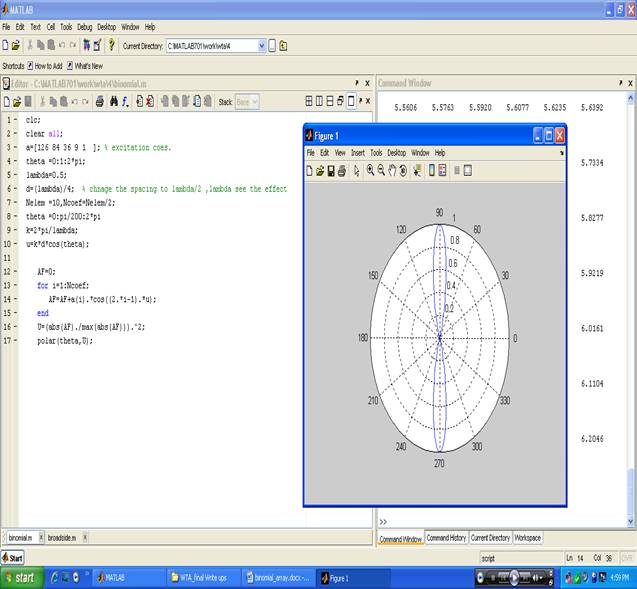Conclusion:

In binomial array, array is arranged in such a way that broadside array radiate more strongly at centre than that from the edges. In order to reduce minor lobe as compared to main lobe i.e. in binomial array no side lobe are present.

Upon completion of experiment students will be able to:

Students will able to model the Binomial array, compute the performance parameters and compare the performance parameters with standard parameters

Oral Question Bank

Theory Question Bank

 Q. No Description 1. What is an â€œarray factorâ€? 2. What is a binomial array? 3. What is the disadvantage of a binomial array? 4. What are the types of antenna arrays? 5. Define the length of an array 6. What are the applications of arrays? 7. Define antenna 8. Define Radiation pattern 9. What are the two types of radiation pattern 10. What is the direction of maximum radiation is maximum only in one direction that is in the direction of array axis. 11. What are the advantages of binomial array? 12. What is the need for the Binomial array? 13. How to convert broad side array radiation pattern into unidirectional? 14. Define antenna array. 15. What is an array and mention the various forms of antenna arrays? 16. What is array factor or space factor? 17. What is the relationship between effective aperture and directivity? 18. Write the principle of pattern multiplication? 19. Define Beam solid angle or beam area? 20. Define beam efficiency?